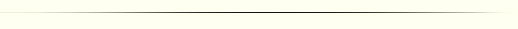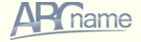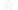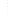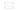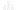ruenuk

##sing in

(044) 362 48 16   (098) 294 41 60Your Online Store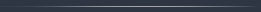WEB developmentServicesPortfolioPricesArticlesProgramming in PHPScripting Java, CGI, network protocolsFundamentals of language htmlTo help the userArticle on the usability of sitesOther topicsServices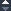Flash developmentPrintingMultimediaRegister domain namesHostingSearch engine optimization and advertisingTechnical support and supportContacts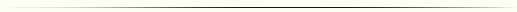### Actually breakdown, or rather arithmetic, 3rd Class

<? php
\$ showperpage
= 0;
ff8000 "> / / how many to show on page
if (isset (\$ HTTP_GET_VARS [ 'show'])) (
0bb "> \$ showperpage = (int) \$ HTTP_POST_VARS [ 'show'];
)
if (isset (\$
HTTP_POST_VARS 700 "> [ 'show'])) (
\$ showperpage = (int) \$ HTTP_POST_VARS [ 'show'7700 ">];
)

/ / How many to display on the page by default
if ((\$ showperpage <1) | | (\$ Showperpage> 100)) (
\$ showperpage = 20 )

/ / How many records get
\$ counted = mysql_num_rows ( color = "# 0000bb"> \$ result);
/ / How many pages will be
\$ countedpages = ceil= "# 007700"> (\$ counted / \$ showperpage);

/ / Get the URL of the page
\$ currentpage = 0;
if (isset (\$
HTTP_GET_VARS [ 'page' \$ currentpage = (int) \$ HTTP_GET_VARS [ 'page'];
& n
bsp;)
if (\$ currentpage> \$ countedpages) (
\$ currentpage = \$ countedpages;
)
if (\$
currentpage <1 font>) (
\$ currentpage = 1;
)

/ / first entry
\$ start_pos = (\$ currentpage - 1) * \$ showperpage # 007700 "> + 1;
/ / Last position
\$ end_pos = \$ start_pos +r = "# 0000bb"> \$ showperpage - 1;
if (\$ end_pos>
\$ counted) (
& nb
sp; \$ end_pos = \$ counted;
)
>
<p> found: <? = \$ counted?> </ p>
<? = \$ currentpage?>
from <? = \$ countedpages?> </ p>
<p> <? php
/ / Display the page to select the
& nb
sp; for (\$ i = 1; \$ i <= \$ countedpages; \$ I + +) (
if (\$ currentpage!
= \$ i) (
& nb
sp; echo "<a href = " ". \$ PHP_SELF."? show = ". \$showperpage. "& page =". \$ i. ""> ". \$ i nt>. "</ a>";
) Else (
echo
\$ i;
)
&
nbsp; echo "";
)

?> </ P>
<? php
if (! mysql_num_rows (\$ result)) (
echo
"<p> Upon request, nothing was found </ p>";
) Else (
& n
bsp; \$ i = \$ start_pos;
echo
"<ol start = " "700 ">. \$ I." ">";
/ / Move to the start position
mysql_data_seek (\$ result, \$ i - 1) or echo "Could not seek to row ". (\$ i - 1);
/ / Display the results found up to the position of \$ end_pos
while (\$ row = mysql_fetch_array (\$ result)) (
& nb
sp; if (\$ i> \$ end_pos) (
break;
)
echo
00 ">" <li> ". \$ Row [ 'field1']." </ Li> ";
&
nbsp; \$ i + +;
)
echo
"</ ol>";
)
>
<br />

### Effectiveness

As you can see in the code set is not an effective concept. First, we return all the results from the SQL query, and then doing the navigation in the results. In terms of the correct approach is ineffective stretch all art years and then remove only the necessary. MySQL allows a further stage of writing the SQL query to limit the result of only the current page through instruction LIMIT. For example to display the 3rd page of 20 lines is enough to write

000bb "> <? Php
\$ result = mysql_query ( "SELECT * FROM table WHERE .... LIMIT 40, 20);
while
(\$ Row = mysql_fetch_array (\$ result)) (
echo
\$ row ['field1'];
)
>

but then the problem is counting all the pages. How do I know how many would back the request records without limiting the LIMIT? Exit the two:

• IfYou were lucky with the version of MySQL> 4.0.x it is:

<? php
\$ sql
= "SELECT SQL_CALC_FOUND_ROWS * FROM table WHERE & n
bsp ;..... LIMIT 0, 200;
\$ result = mysql_query (\$ sql);
ont> \$ sql = "SELECT FOUND_ROWS ()";
\$ count_row = mysql_fetch_rowolor = "# 007700"> (mysql_query (\$ sql));
\$ counted = \$ count_row ;
>

• Pre-do SELECT COUNT (id) FROM table WHERE ...

Will these options more effectively in your particular case, decide you experiencedm through. With the new MySQL version probably will be better option as a separate calculation would be acceptable only in the case of simple sampling WHERE index. The last option, for example, implemented in phpMyAdmin

 Lock files 29.05.2007 How to send e-mail 29.05.2007 Lock files 29.05.2007